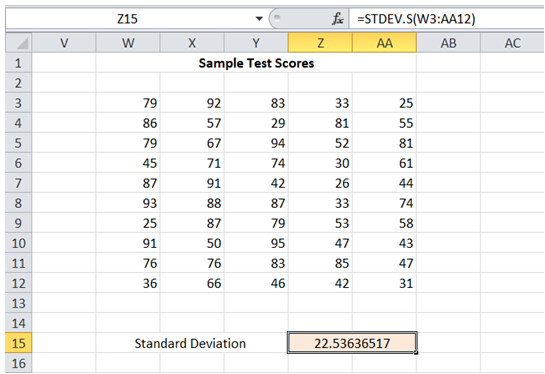## Standard deviation range in Excel

For example, I have test scores of 50 students drawn from a population of 2000 students that took an exam. I want to get an estimate of Standard Deviation.

To do it in Excel, here is the answer:

a) Enter formula =STDEV.S(W3:AA12) where W3:AA12 corresponds to the range with Test Scores.

In case the 50 Test scores represent the entire population, Standard Deviation for the population can be calculated by substituting STDEV.S with STDEV.P.